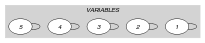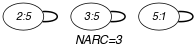## 5.295. open_among

Origin
Constraint

$\mathrm{𝚘𝚙𝚎𝚗}_\mathrm{𝚊𝚖𝚘𝚗𝚐}\left(𝚂,\mathrm{𝙽𝚅𝙰𝚁},\mathrm{𝚅𝙰𝚁𝙸𝙰𝙱𝙻𝙴𝚂},\mathrm{𝚅𝙰𝙻𝚄𝙴𝚂}\right)$

Arguments
 $𝚂$ $\mathrm{𝚜𝚟𝚊𝚛}$ $\mathrm{𝙽𝚅𝙰𝚁}$ $\mathrm{𝚍𝚟𝚊𝚛}$ $\mathrm{𝚅𝙰𝚁𝙸𝙰𝙱𝙻𝙴𝚂}$ $\mathrm{𝚌𝚘𝚕𝚕𝚎𝚌𝚝𝚒𝚘𝚗}\left(\mathrm{𝚟𝚊𝚛}-\mathrm{𝚍𝚟𝚊𝚛}\right)$ $\mathrm{𝚅𝙰𝙻𝚄𝙴𝚂}$ $\mathrm{𝚌𝚘𝚕𝚕𝚎𝚌𝚝𝚒𝚘𝚗}\left(\mathrm{𝚟𝚊𝚕}-\mathrm{𝚒𝚗𝚝}\right)$
Restrictions
 $𝚂\ge 1$ $𝚂\le |\mathrm{𝚅𝙰𝚁𝙸𝙰𝙱𝙻𝙴𝚂}|$ $\mathrm{𝙽𝚅𝙰𝚁}\ge 0$ $\mathrm{𝙽𝚅𝙰𝚁}\le |\mathrm{𝚅𝙰𝚁𝙸𝙰𝙱𝙻𝙴𝚂}|$ $\mathrm{𝚛𝚎𝚚𝚞𝚒𝚛𝚎𝚍}$$\left(\mathrm{𝚅𝙰𝚁𝙸𝙰𝙱𝙻𝙴𝚂},\mathrm{𝚟𝚊𝚛}\right)$ $\mathrm{𝚛𝚎𝚚𝚞𝚒𝚛𝚎𝚍}$$\left(\mathrm{𝚅𝙰𝙻𝚄𝙴𝚂},\mathrm{𝚟𝚊𝚕}\right)$ $\mathrm{𝚍𝚒𝚜𝚝𝚒𝚗𝚌𝚝}$$\left(\mathrm{𝚅𝙰𝙻𝚄𝙴𝚂},\mathrm{𝚟𝚊𝚕}\right)$
Purpose

Let $𝒱$ be the variables of the collection $\mathrm{𝚅𝙰𝚁𝙸𝙰𝙱𝙻𝙴𝚂}$ for which the corresponding position belongs to the set $𝚂$. Positions are numbered from 1. $\mathrm{𝙽𝚅𝙰𝚁}$ is the number of variables of $𝒱$ that take their value in $\mathrm{𝚅𝙰𝙻𝚄𝙴𝚂}$.

Example
$\left(\left\{2,3,4,5\right\},3,〈8,5,5,4,1〉,〈1,5,8〉\right)$

The $\mathrm{𝚘𝚙𝚎𝚗}_\mathrm{𝚊𝚖𝚘𝚗𝚐}$ constraint holds since within the last four values (i.e., $𝚂=\left\{2,3,4,5\right\}$) of $〈8,5,5,4,1〉$ exactly 3 values belong to the set of values $\left\{1,5,8\right\}$.

Typical
 $\mathrm{𝙽𝚅𝙰𝚁}>0$ $\mathrm{𝙽𝚅𝙰𝚁}<|\mathrm{𝚅𝙰𝚁𝙸𝙰𝙱𝙻𝙴𝚂}|$ $|\mathrm{𝚅𝙰𝚁𝙸𝙰𝙱𝙻𝙴𝚂}|>1$ $|\mathrm{𝚅𝙰𝙻𝚄𝙴𝚂}|>1$ $|\mathrm{𝚅𝙰𝚁𝙸𝙰𝙱𝙻𝙴𝚂}|>|\mathrm{𝚅𝙰𝙻𝚄𝙴𝚂}|$
Symmetries
• Items of $\mathrm{𝚅𝙰𝙻𝚄𝙴𝚂}$ are permutable.

• An occurrence of a value of $\mathrm{𝚅𝙰𝚁𝙸𝙰𝙱𝙻𝙴𝚂}.\mathrm{𝚟𝚊𝚛}$ that belongs to $\mathrm{𝚅𝙰𝙻𝚄𝙴𝚂}.\mathrm{𝚟𝚊𝚕}$ (resp. does not belong to $\mathrm{𝚅𝙰𝙻𝚄𝙴𝚂}.\mathrm{𝚟𝚊𝚕}$) can be replaced by any other value in $\mathrm{𝚅𝙰𝙻𝚄𝙴𝚂}.\mathrm{𝚟𝚊𝚕}$ (resp. not in $\mathrm{𝚅𝙰𝙻𝚄𝙴𝚂}.\mathrm{𝚟𝚊𝚕}$).

Arg. properties
• Functional dependency: $\mathrm{𝙽𝚅𝙰𝚁}$ determined by $𝚂$, $\mathrm{𝚅𝙰𝚁𝙸𝙰𝙱𝙻𝙴𝚂}$ and $\mathrm{𝚅𝙰𝙻𝚄𝙴𝚂}$.

• Suffix-contractible wrt. $\mathrm{𝚅𝙰𝚁𝙸𝙰𝙱𝙻𝙴𝚂}$ when $\mathrm{𝙽𝚅𝙰𝚁}=0$.

Keywords
Arc input(s)

$\mathrm{𝚅𝙰𝚁𝙸𝙰𝙱𝙻𝙴𝚂}$

Arc generator
$\mathrm{𝑆𝐸𝐿𝐹}$$↦\mathrm{𝚌𝚘𝚕𝚕𝚎𝚌𝚝𝚒𝚘𝚗}\left(\mathrm{𝚟𝚊𝚛𝚒𝚊𝚋𝚕𝚎𝚜}\right)$

Arc arity
Arc constraint(s)
 $•$$\mathrm{𝚒𝚗}$$\left(\mathrm{𝚟𝚊𝚛𝚒𝚊𝚋𝚕𝚎𝚜}.\mathrm{𝚟𝚊𝚛},\mathrm{𝚅𝙰𝙻𝚄𝙴𝚂}\right)$ $•$$\mathrm{𝚒𝚗}_\mathrm{𝚜𝚎𝚝}$$\left(\mathrm{𝚟𝚊𝚛𝚒𝚊𝚋𝚕𝚎𝚜}.\mathrm{𝚔𝚎𝚢},𝚂\right)$
Graph property(ies)
$\mathrm{𝐍𝐀𝐑𝐂}$$=\mathrm{𝙽𝚅𝙰𝚁}$

Graph model

The arc constraint corresponds to the conjunction of unary constraints $\mathrm{𝚒𝚗}\left(\mathrm{𝚟𝚊𝚛𝚒𝚊𝚋𝚕𝚎𝚜}.\mathrm{𝚟𝚊𝚛},\mathrm{𝚅𝙰𝙻𝚄𝙴𝚂}\right)$ and $\mathrm{𝚒𝚗}_\mathrm{𝚜𝚎𝚝}\left(\mathrm{𝚟𝚊𝚛𝚒𝚊𝚋𝚕𝚎𝚜}.\mathrm{𝚔𝚎𝚢},𝚂\right)$ defined in this catalogue. Consequently we employ the $\mathrm{𝑆𝐸𝐿𝐹}$ arc generator in order to produce an initial graph with a single loop on each vertex.

Parts (A) and (B) of Figure 5.295.1 respectively show the initial and final graph associated with the Example slot. Since we use the $\mathrm{𝐍𝐀𝐑𝐂}$ graph property, the loops of the final graph are stressed in bold.

##### Figure 5.295.1. Initial and final graph of the $\mathrm{𝚘𝚙𝚎𝚗}_\mathrm{𝚊𝚖𝚘𝚗𝚐}$ constraint(a) (b)